TL;DR: This is an informal summary of our recent article Sparser Kernel Herding with Pairwise Conditional Gradients without Swap Steps by Kazuma Tsuji, Ken’ichiro Tanaka, and Sebastian Pokutta, which was accepted at ICML 2022. In this article we present a modification of the pairwise conditional gradient algorithm that removes the dreaded swap steps. The resulting algorithm can even be applied to infinite dimensional feasible regions and promotes high levels of sparsity, which is useful in applications such as e.g., kernel herding.

Written by Sebastian Pokutta.

## What is the paper about and why you might care

One of the most appealing conditional gradient algorithm is the Pairwise Conditional Gradient (PCG) algorithm introduced in [LJ] as a modification of the Away-step Frank-Wolfe (AFW) algorithm. The Pairwise Conditional Gradient algorithm essentially performs a normal Frank-Wolfe step and an away-step simultaneously. This gives much higher convergence steps in practice, however in the analysis now, so-called swap steps appear, where weight is shifted from an away-vertex to a new Frank-Wolfe vertex. For these steps, we cannot bound the primal progress and additionally, there are potentially lots of these steps, so that the theoretical convegence bounds are much worse than what we observe in practice. In fact its guarantees are worse than the guarantees for the Away-step Frank-Wolfe algorithm, that is almost always outperformed by the Pairwise Conditional Gradient algorithm in practice. Various modifications of PCG had been suggested, e.g., in [RZ] and [MGP] to deal with this issue, however often requiring subprocedures whose costs cannot be easily bounded.

It should also be mentioned that the PCG algorithm is particularly nice in the case of polytopes and almost becomes a combinatorial algorithm as the resulting directions are always formed by direction arising from the line-segment between two vertices and thus there are only finitely many such directions.

## Our results

By borrowing machinery from [BPTW], we show that with a minor modification of the PCG algorithm we can avoid drop steps altogether. In a nutshell, the idea is to limit the pairwise directions to those formed by FW vertices and away-vertices from the current active set and only if those steps are not good enough we perform a normal FW step. This way swap steps cannot appear anymore however we require a key technical lemma that shows that the reduced pairwise steps are still good enough. We call this algorithm the Blended Pairwise Conditional Gradient (BPCG) algorithm; see below.

The resulting algorithm has a theoretical convergence rate that is basically the same as the one for the AFW algorithm (up to small constant factors). In fact, it inherits all convergence proofs that hold for the AFW algorithm. Moreover, it exhibits the same convergence speed as (even often faster than) the original PCG algorithm in practice. The algorithm also works in the infinite dimensional setting, which is not true for the original PCG algorithm due to the dimension dependence arising from the number of swap steps in the convergence rate. The iterates produced by BPCG are very sparse, where sparsity is measured in the number of elements in the convex combinations of the iterates making it very suitable, e.g., for kernel herding.

Blended Pairwise Conditional Gradient Algorithm (BPCG); slightly simplified
Input: Smooth convex function $f$ with first-order oracle access, feasible region (polytope) $P$ with linear optimization oracle access, initial vertex $x_0 \in P$.
Output: Sequence of points $x_0, \dots, x_{T}$
$$S_0 = \{x_0\}$$
For $t = 0, \dots, T-1$ do:
$\quad a_t \leftarrow \arg\max_{v \in S_t} \langle \nabla f(x_{t}), v \rangle$ $\qquad$ {Away-vertex over $S_t$}
$\quad s_t \leftarrow \arg\min_{x \in S_t} \langle \nabla f(x_{t}), v \rangle$ $\qquad$ {Local FW-vertex over $S_t$}
$\quad w_t \leftarrow \arg\min_{x \in P} \langle \nabla f(x_{t}), v \rangle$ $\qquad$ {(Global) FW-vertex over $P$}
$\quad$ If $$\langle \nabla f(x_{t}), a_t - s_t \rangle \geq \langle \nabla f(x_{t}), x_t - w_t \rangle$$: $\qquad$ {Local gap as large as global gap}
$\quad\quad$ $d_t = a_t - s_t$ $\qquad$ {Pick (local) pairwise direction}
$\quad\quad$ $x_{t+1} \leftarrow x_t - \gamma_t d_t$ via line search s.t. residual weight of $a_t$ nonnegative
$\quad\quad$ If $a_t$ removed then {Drop step} $$S_{t+1} \leftarrow S_t \setminus \{a_t\}$$ else {Descent step} $$S_{t+1} \leftarrow S_t$$
$\quad$ Else $\qquad$ {Normal FW Step}
$\quad\quad$ $d_t = x_t - w_t$ $\qquad$ {Pick FW direction}
$\quad\quad$ $x_{t+1} \leftarrow x_t - \gamma_t d_t$ via line search with $\gamma_t \in [0,1]$
$\quad\quad$ Update $$S_{t+1} \leftarrow S_t \cup \{w_t\}$$

The key to the convergence proofs is the following lemma that show that these local pairwise steps combined with global FW steps are good enough to ensure sufficient progress per iteration

Key Lemma. In each iteration $t$ it holds: $2 \langle \nabla f(x_{t}), d_t \rangle \geq \langle \nabla f(x_{t}), a_t - w_t \rangle.$

For those in the know this is all you need to prove convergence: the term on the right-hand side is the strong Wolfe gap and as such progress from smoothness with $d_t$ can be lower bounded by the progress from smoothness with the strong Wolfe gap. See e.g., Cheat Sheet: Smooth Convex Optimization and Cheat Sheet: Linear convergence for Conditional Gradients (towards the end when analyzing AFW) to understand how one continues from here. Also similarly, with this inequality we can apply e.g., the reasoning in Cheat Sheet: Hölder Error Bounds (HEB) for Conditional Gradients to extend the results, to e.g., sharp functions etc.

### Computations

We also performed several computational experiments to evaluate the performance of BPCG and in fact the algorithm has been also implemented in the FrankWolfe.jl Julia Package (See this post or [BCP]) and is now the recommended default active-set based conditional gradient algorithm. With a simple trick by adding a factor on the left-hand side in front of the gap test in the algorithm one can further improve sparsity; see the original paper for more details.

Below in Figure 1, we provide a simple convergence test for the approximate Carathéodory problem. We can see that in iterations BPCG is basically identical to PCG (as expected) however in time it is faster as the local updates are often cheaper.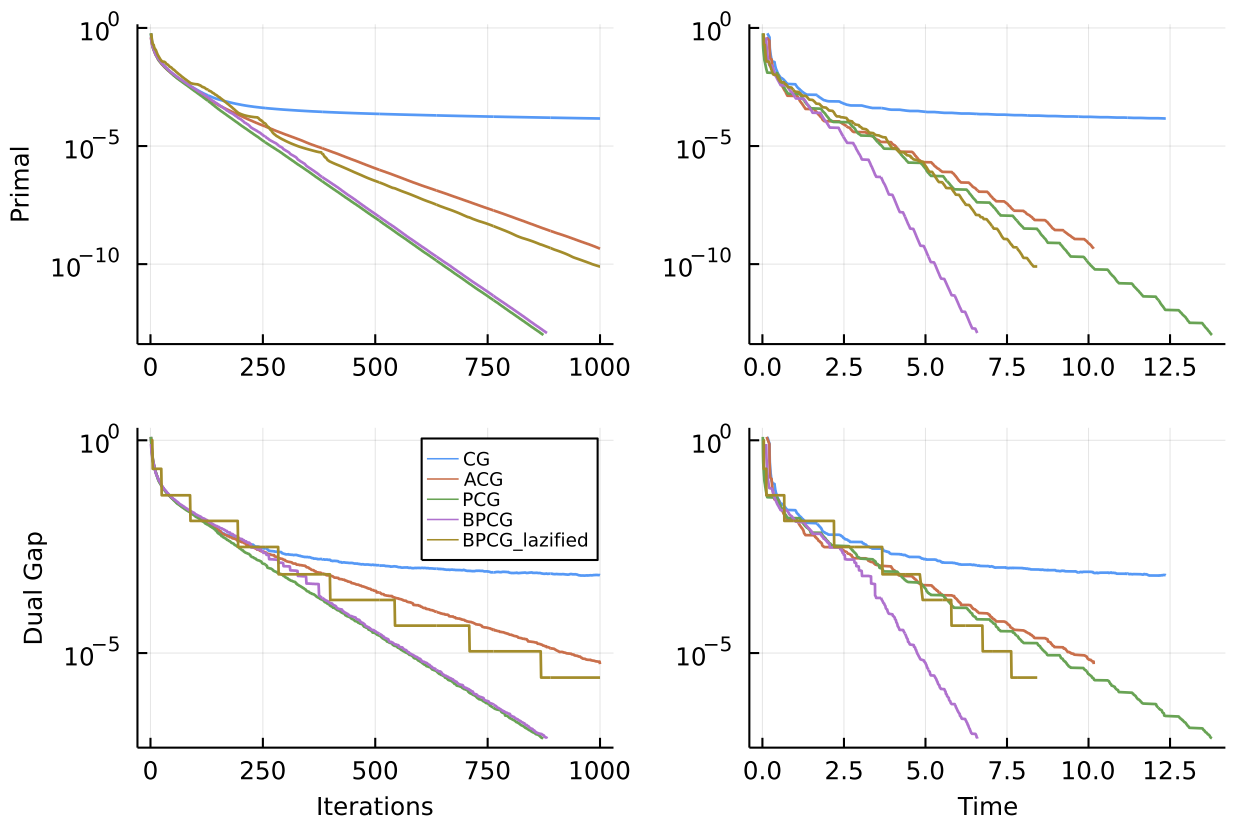Figure 1. Convergence on approximate Carathéodory instance over polytope of dimension $n=200$.

#### Sparsity

While the convergence plot above is quite typical and in terms of speed per iteration or wallclock time BPCG is usually at least as good as PCG (and sometimes faster), the real advantage is often in terms of sparsity as the preference for local steps promotes sparsity. This can be seen in the two plots below.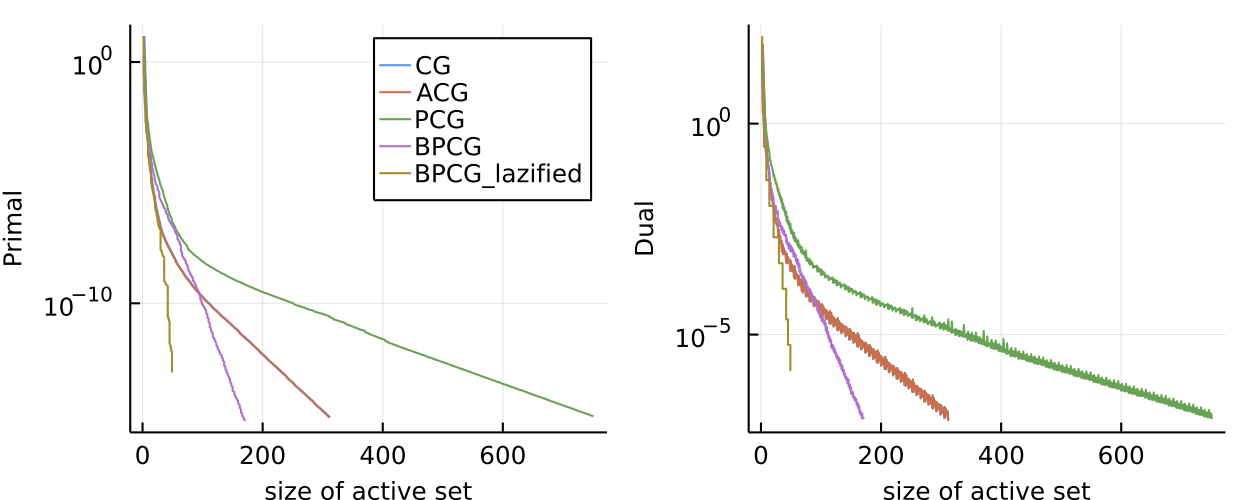Figure 2. Sparse regression problem over $l_5$-norm ball. Here we plot primal value and dual gap vs. size of the active set. BPCG consistently delivers smaller primal and dual values for the same number of atoms in the active set.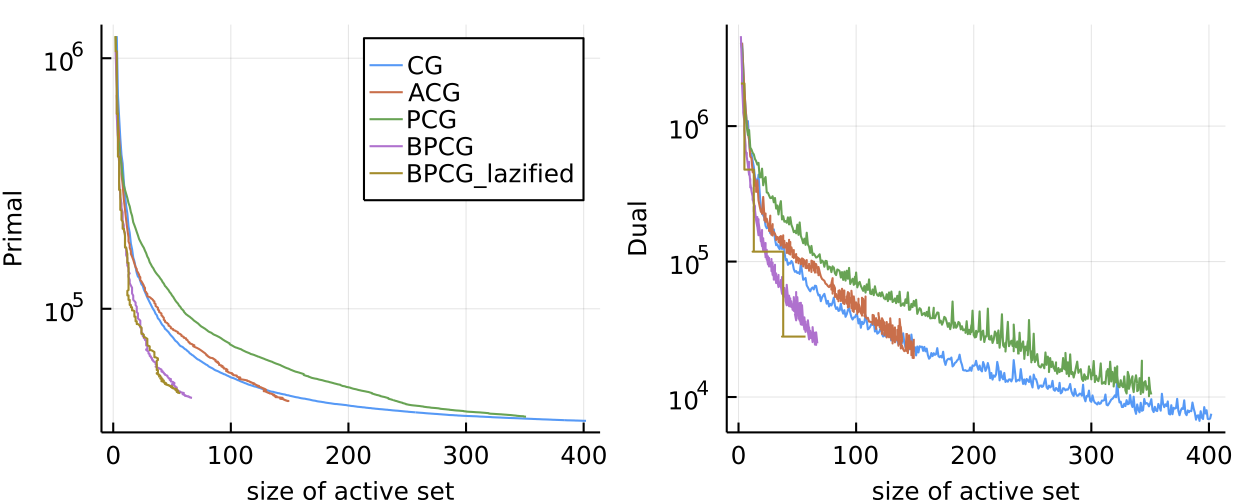Figure 3. Movielens matrix completion problem. Same logic as above with similar results.

#### Kernel Herding

Finally we also considered various kernel herding problems. Here are two examples; the graphs are a little packed.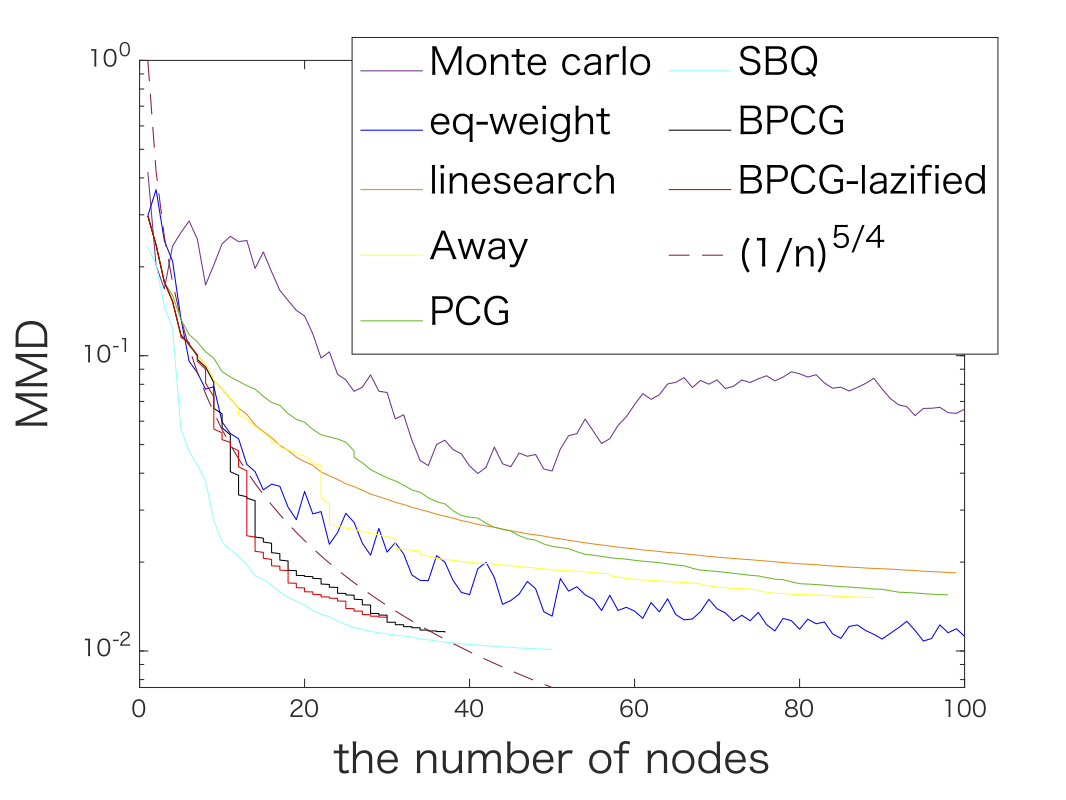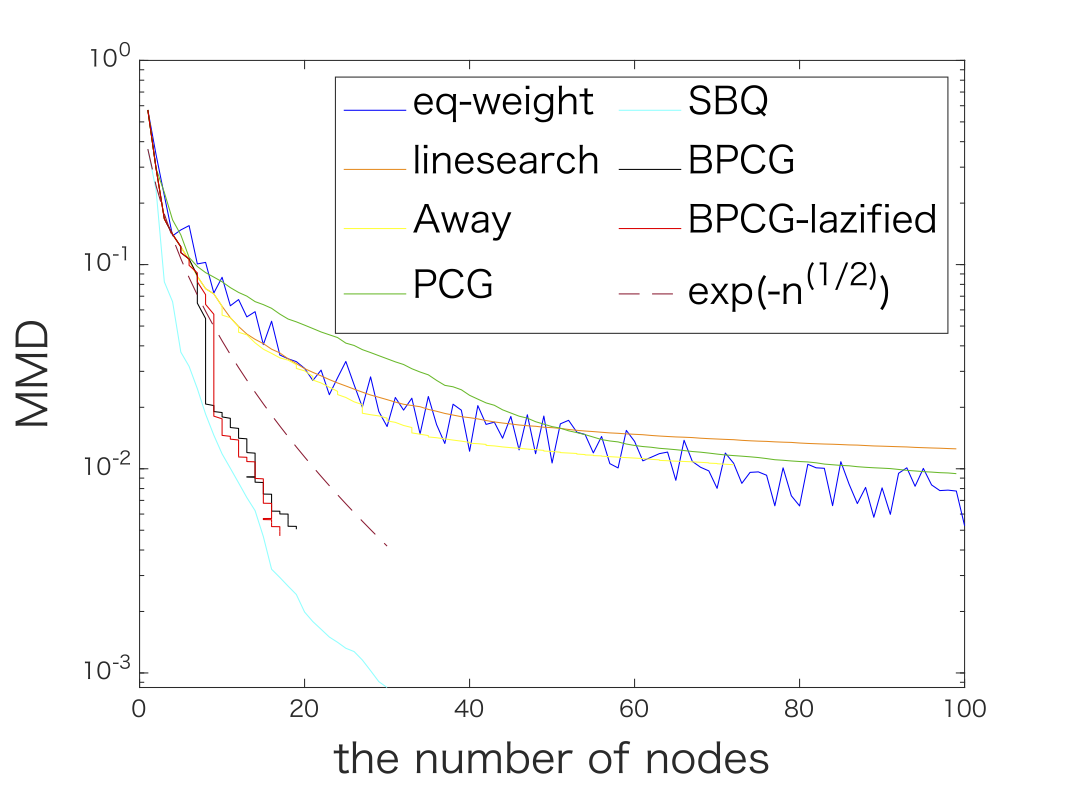Figure 4. Kernel herding for Matérn kernel (left) and Gaussian kernel (right). In both cases BPCG delivers results on par with the Sequential Bayesian Quadrature method (SBQ) however at the fraction of the cost.

[LJ] Lacoste-Julien, S., & Jaggi, M. (2015). On the global linear convergence of Frank-Wolfe optimization variants. In Advances in Neural Information Processing Systems (pp. 496-504). pdf

[RZ] Rinaldi, F., & Zeffiro, D. (2020). A unifying framework for the analysis of projection-free first-order methods under a sufficient slope condition. arXiv preprint arXiv:2008.09781. pdf

[MGP] Mortagy, H., Gupta, S., & Pokutta, S. (2020). Walking in the shadow: A new perspective on descent directions for constrained minimization. Advances in Neural Information Processing Systems, 33, 12873-12883. pdf

[BPTW] Braun, G., Pokutta, S., Tu, D., & Wright, S. (2019, May). Blended conditonal gradients. In International Conference on Machine Learning (pp. 735-743). PMLR. pdf

[BCP] Besançon, M., Carderera, A., & Pokutta, S. (2022). FrankWolfe. jl: A High-Performance and Flexible Toolbox for Frank–Wolfe Algorithms and Conditional Gradients. INFORMS Journal on Computing. pdf